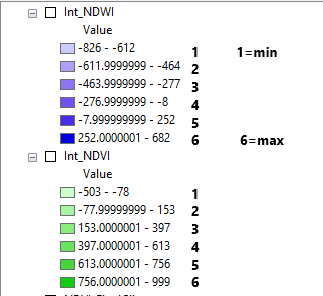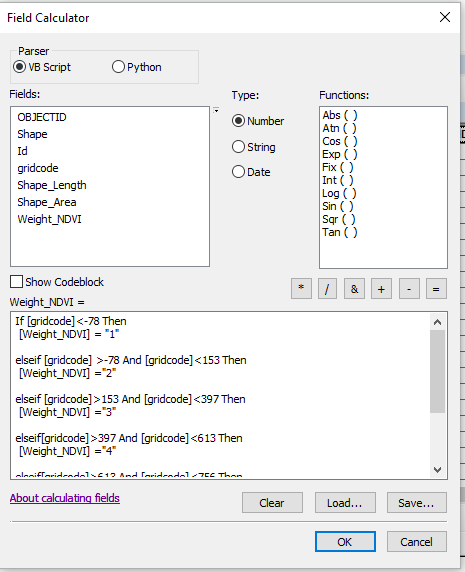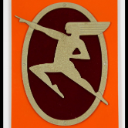# VBscript to group discrete integer polygon gridcodes into natural breaks using the Field Calculator

214
3
11-22-2019 08:51 AMNew Contributor

The goal was to use the weighted overlay tool to create a wetland suitability index using 3 indices: soil conditions, NDVI, and NDWI.  Originally the NDVI and NDWI were integer rasters but due to the soil map being a vector file i choose to convert the rasters to vector and go about weighting them using the Field Calculator.   I would have used the weighted overlay but due to the wide range of values assigning every single scale value is a waste of time in my opinion.

The idea was to emulate the natural breaks in the symbology as the groupings that would be assigned scale value as such:scale values range from 1-6, 1 = the lowest suitability and 6 = maximum suitability

@@

I have little to no experience with VBscript or Python so here is what i came up with referencing this page: Calculate Field examples—Help | ArcGIS Desktop

but due to syntax errors it doesn't work.  Hopefully someone can point me in the right direction or explain why the script is not working as intended.   Here is a screenshot of the Field Calculator and the entire codeblockExpression:

Weight_NDVI

Code Block:

If [gridcode]<-78 Then
[Weight_NDVI] = "1"

elseif [gridcode] >-78 And [gridcode]<153 Then
[Weight_NDVI] ="2"

elseif [gridcode]>153 And [gridcode]<397 Then
[Weight_NDVI] ="3"

elseif [gridcode]>397 And [gridcode]<613 Then
[Weight_NDVI] ="4"

elseif [gridcode]>613 And [gridcode]<756 Then
[Weight_NDVI] ="5"

elseif [gridcode]>756 And [gridcode]<999 Then
[Weight_NDVI] ="6"

end if

Any help is much appreciated, even if it's only a forum link to help me figure it out myself!  Thanks!

Tags (2)
1 Solution

Accepted SolutionsbyMVP Honored Contributor

First, click the "Show Codeblock" option. Use this in the "Pre-Logic Script Code" area

``````dim temp
If [gridcode]<-78 Then
temp = 1
elseif [gridcode] < 153 Then
temp = 2
elseif [gridcode] < 397 Then
temp = 3
elseif [gridcode] < 613 Then
temp = 4
elseif [gridcode] < 756 Then
temp = 5
elseif [gridcode] < 999 Then
temp = 6
end if‍‍‍‍‍‍‍‍‍‍‍‍‍‍‍‍‍‍‍‍‍‍‍‍‍‍‍‍‍‍‍‍‍‍‍‍‍‍‍‍‍‍‍‍‍‍‍‍‍‍‍‍‍‍‍‍‍‍‍‍‍‍‍‍‍‍‍‍‍‍‍‍‍‍‍‍‍‍‍‍‍‍``````

and put this in the "[Weight_NDVI] ="

``temp‍‍``
3 RepliesbyMVP Honored Contributor

Is "Weight_NDVI" a string or numeric field?New Contributor

Numeric, i just created a new field in the attribute table, type: doublebyMVP Honored Contributor

First, click the "Show Codeblock" option. Use this in the "Pre-Logic Script Code" area

``````dim temp
If [gridcode]<-78 Then
temp = 1
elseif [gridcode] < 153 Then
temp = 2
elseif [gridcode] < 397 Then
temp = 3
elseif [gridcode] < 613 Then
temp = 4
elseif [gridcode] < 756 Then
temp = 5
elseif [gridcode] < 999 Then
temp = 6
end if‍‍‍‍‍‍‍‍‍‍‍‍‍‍‍‍‍‍‍‍‍‍‍‍‍‍‍‍‍‍‍‍‍‍‍‍‍‍‍‍‍‍‍‍‍‍‍‍‍‍‍‍‍‍‍‍‍‍‍‍‍‍‍‍‍‍‍‍‍‍‍‍‍‍‍‍‍‍‍‍‍‍``````

and put this in the "[Weight_NDVI] ="

``temp‍‍``